# Solving Mixed Operations Word Problems

Knowing what operation to use in a mixed word problem can be tricky for students. They easily get bogged down in the numbers before they think about which operation should be used.

## Remove the numbers to focus on the operations

One way to solve this is to remove the numbers from the word problem to focus on the operations first. Let us show you how in an example.

A warehouse received a delivery of 12 crates of bananas. There were 42 bananas in each crate. 4 bananas were rotten and had to be thrown away. The remaining bananas were packed into boxes of 10 to be delivered to the store. How many boxes of bananas were delivered to the store?

Now, let’s remove the numbers to focus on the operations to be completed:

A warehouse received a delivery of ___ crates of bananas. There were ___ bananas in each crate. ___ bananas were rotten and had to be thrown away. The remaining bananas were packed into boxes of ___ to be delivered to the store. How many boxes of bananas were delivered to the store?

First, we want to work out how many bananas were received by the warehouse.

We multiply the crates of bananas by the bananas in each crate. That’s the first two sentences taken care of.

Next, we need to remove the rotten bananas.

We take the total number of bananas and subtract the rotten bananas. That’s the third sentence taken care of.

Then, we want to work out how many boxes are used to re-pack the bananas.

We take the total number of good bananas and divide that number by 10 (as there are 10 bananas in each new box).

So, the operations we will complete are:

Multiplication

Subtraction

Division

Now, let’s apply those to the example.

12 (crates) x 42 (bananas) = 504 bananas

504 (bananas) – 4 (rotten bananas) = 500 bananas

500 bananas / 10 bananas = 50 boxes

There were 50 boxes of bananas delivered to the store.

## Use block diagrams to illustrate the word problem

Another way is to use block diagrams. Let’s use the same example.

First, we want to work out how many bananas were received by the warehouse.

We multiply the crates of bananas by the bananas in each crate.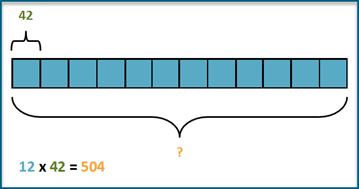Next, we need to remove the rotten bananas.

We take the total number of bananas and subtract the rotten bananas.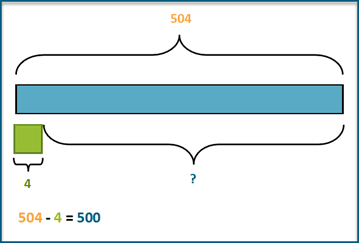Then, we want to work out how many boxes are used to re-pack the bananas.

We take the total number of good bananas and divide that number by 10 (as there are 10 bananas in each new box).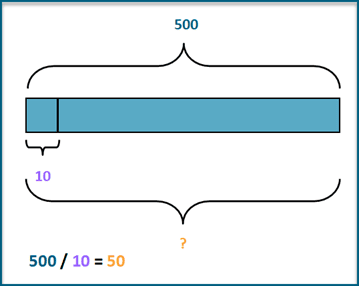There were 50 boxes of bananas delivered to the store.

## Grade 3 mental math mixed word problems

We’ve created a set of mixed word problems for grade 3 students that allow them to use mental math.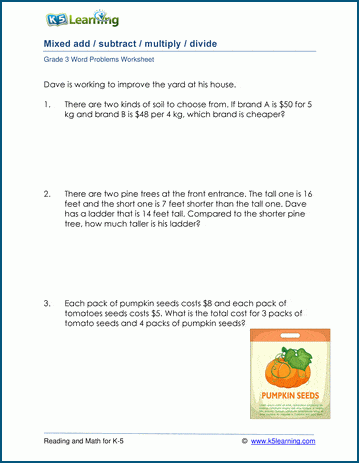## Mixed word problems using column math for grade 3

With this set of harder mixed word problems worksheets, students use column math to solve the problems.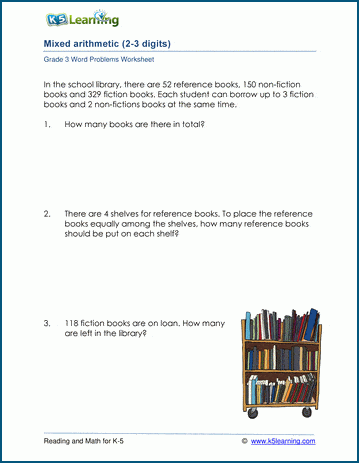## Mixed word problems for grade 4

These four operations word problems are intended for grade 4 students.